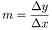# Glossar

All · A · C · D · E · F · G · L · M · N · P · S · T · W · Z

# L

## Linear Functions

Linear functions show proportional dependencies. This means that the ratio of two quantities to each other always remains the same. The function graph is therefore a straight line.

The general form is: $f\left(x\right)=mx+n$. In this equation, $m$ is the gradient, calculated from,
and $n$ is the intercept of the graph with the y-axis.